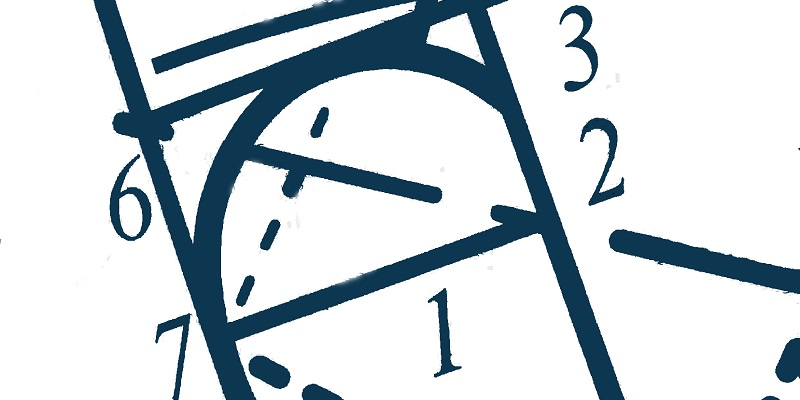# Logic

## Our researchOur members and their current research interests include:

### Dr Andrew Brooke-Taylor

Works in set theory, with an emphasis on applications to category theory, algebraic topology and other related areas.  He is particularly interested in large cardinal axioms and forcing, with ongoing research interests in cardinal characteristics at large cardinals, connections between Borel reducibility and universal categories, relative consistency results around the level of Vopenka’s Principle, and applications of large cardinals in the theory of accessible categories.

### Dr Pantelis Eleftheriou

Works in model theory and its connections to algebra, geometry and number theory. His main interest is to recover concrete mathematical structure from given logical data, such as Lie groups from definable groups, algebraic curves from definable sets with many rational points, algebraically closed fields from strongly minimal structures, and algebraic topology (homotopy/homology) from semi-linear data. He has recently become interested in applications of model theory to computer science, combinatorics, and the Vapnik-Chervonenkis theory in machine learning.

### Professor Dugald Macpherson

Works in model theory and its connections to algebra and combinatorics. Particular areas of current interest are: permutation groups (usually infinite), typically automorphism groups of countable first order structures; model theory of groups; model theory of families of finite structures (e.g. finite fields, finite simple groups, classes of finite graphs) and their infinite limits; model theory of fields (e.g. valued fields); abstract model theory around stability theory and its generalisations, and connections to combinatorics.

### Dr Vincenzo Mantova

Interests are around the model theory of exponentiation and of other analytic functions. His main focus is on taming complex exponentiation and other exponential functions on complex Lie groups, along the strategy set up by Zilber, and fast growing real functions, via surreal numbers and transseries. He also has an interest in Diophantine geometry, especially in connection with the aforementioned problems.

### Professor Michael Rathjen

Main interests are in proof theory (cut elimination for infinitary proof systems; ordinal analysis of classical and intuitionistic theories; witness extraction from proofs), intuitionism and constructive mathematics (frameworks for constructivism: constructive set theory, explicit mathematics, Martin-Löf type theory; realizability and forcing techniques), mostly non-classical set theory (proof theory and ordinal analysis of set theories; admissible set theory; constructive and intuitionistic set theory; set theory with anti-foundation axiom; 'large cardinals' axioms in constructive and intuitionistic set theories), reverse mathematics and combinatorial principles (Kruskal's Theorem, Graph Minor Theorem, …), philosophy of mathematics.

### Dr Paul Shafer

Works in computability theory, with an eye to describing the complexities of mathematical problems. He is particularly interested in reverse mathematics and in the Medvedev and Muchnik degrees.  Reverse mathematics is an analysis of the logical strengths of mathematical theorems in the context of second-order arithmetic. Here Paul studies theorems from algebra, analysis, combinatorics, logic itself, and topology. The Medvedev and Muchnik degrees are higher-order analogues of the Turing degrees designed to model the “problem A is computationally harder than problem B” relation. Here Paul studies the algebraic properties of these degree structures, their complexities, and their uses as computational semantics for various logics.

### Professor John K Truss

Main interests are axiomatic set theory, specifically questions about the axiom of choice, infinite permutation groups, and their connections with model theory. Three recent pieces of work concern automatic homeomorphicity, Ehrenfeucht-Fraïssé games between linearly ordered sets, and constructions of rigid chains. A structure is said to have automatic homeomorphicity if any isomorphism from its endomorphism monoid to the full transformation monoid of a countable set is necessarily also a homeomorphism. This has been verified for monoids derived from the rationals and its reducts, and also associated clones. Ehrenfeucht-Fraïssé games between two structures provide a good way of analysing how similar the two structures are. This has been done for various classes of linear orders, sometimes with colours. A classical construction of Dushnik and Miller of a rigid dense subchain of the ordered set of real numbers, where ‘rigid’ means that there are no non- trivial automorphisms, has been generalized to give results also about rigidity for embeddings and epimorphisms. This is also extended to higher cardinalities.